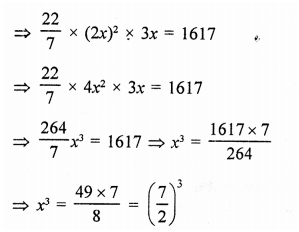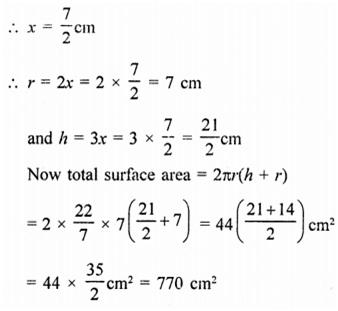• +91 9971497814
• info@interviewmaterial.com

# RD Chapter 19- Surface Areas and Volume of a Circular Cylinder Ex-VSAQS Interview Questions Answers

### Related Subjects

Question 1 : Write the number of surface of a right circular cylinder.

Answer 1 : Three, two circular and one curved.

Question 2 : Write the ratio of total surface area to the curved surface area of a cylinder of radius r and height h.

and height = h
∴ Curved surface area = 2πrh
and total surface area = 2πr(h + r)
∴ Ratio = 2πr(h + r) : 2πrh
= h + r : h

Question 3 : The ratio between the radius of the base and height of a cylinder is 2 : 3. If its volume is 1617 cm3, find the total surface area of the cylinder.

Answer 3 : Ratio in radius and height of the cylinder = 2 : 3
Then height (h) = 3x
Volume = πr2hQuestion 4 : If the radii of two cylinders are in the ratio 2 : 3 and their heights are in the ratio 5 : 3, then find the ratio of their volumes.

Ratio of radii of two cylinder = 2:3
Let radius of first cylinder (r1)= 2x
and second cylinder (r2) = 3x
and ratio in their heights = 5:3
Let height of first cylinder (h1) = 5y
and height of second (h2) = 3y
Volumeof the first cylinder =πr2h
= π x (2x)2 x 5y = 20πx2y
and volume of second cylinder = π(3x)2 x 3y = 27πx2y
Now ratio between then,
= 20πx2y: 21πx2y
= 20 : 27

Todays Deals### RD Chapter 19- Surface Areas and Volume of a Circular Cylinder Ex-VSAQS Contributorskrishan

Name:
Email:

# Latest News# 9000 interview questions in different categories